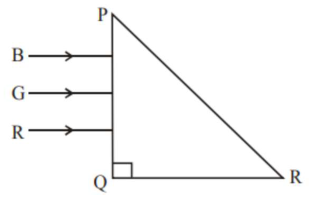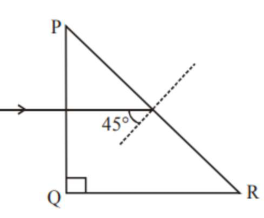# Three rays of light, namely red R,Question:

Three rays of light, namely red $(R)$, green $(G)$ and blue $(B)$ are incident on the face PQ of a right angled prism PQR as shown in figure.The refractive indices of the material of the prism for red, green and blue wavelength are $1.27,1.42$ and $1.49$ respectively. The colour of the ray(s) emerging out of the face $\mathrm{PR}$ is :

1. green

2. red

3. blue and green

4. blue

Correct Option: , 2

Solution:

(2)Assuming that the right angled prism is an isoceles prism, so the other

angles will be $45^{\circ}$ each.

$\Rightarrow$ Each incident ray will make an angle of $45^{\circ}$ with the normal at face PR.

$\Rightarrow$ The wavelength corresponding to which the incidence angle is less than

the critical angle, will pass through $\mathrm{PR} . \Rightarrow \theta_{\mathrm{C}}=$ critical angle

$\Rightarrow \theta_{\mathrm{C}}=\sin ^{-1}\left(\frac{1}{\mu}\right)$

$\Rightarrow$ If $\theta_{C} \geq 45^{\circ}$

the light ray will pass

$\Rightarrow\left(\theta_{\mathrm{C}}\right)_{\operatorname{Red}}=\sin ^{-1}\left(\frac{1}{1.27}\right)=51.94^{\circ}$

Red will pass.

$\Rightarrow\left(\theta_{\mathrm{C}}\right)_{\text {Green }}=\sin ^{-1}\left(\frac{1}{1.42}\right)=44.76^{\circ}$

Green will not pass

$\Rightarrow\left(\theta_{\mathrm{C}}\right)_{\text {Bluc }}=\sin ^{-1}\left(\frac{1}{1.49}\right)=42.15^{\circ}$

Blue will not pass

$\Rightarrow$ So only red will pass through PR.